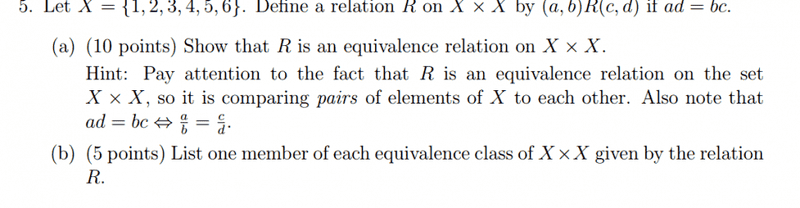# Equivalence relation help....

Kingyou123

## Homework Statementa/b=c/d

## The Attempt at a Solution

I started out with transitivity and I figure it's transitive because a/b=e/f = af=be=(a,b)R(e,f).
It can't be symmetric because ad does not equal bc, 1*4 does not equal 2*3
I'm I correct or completely wrong?

Mentor
2022 Award
Wrong. What does equivalence mean? Can you define all properties? (first in general)

Gold Member
You have to show that the relation R is:

1) Reflexive: (a,b)R(a,b)
2) Symmetric: (a,b)R(c,d) iff (c,d)R(a,b)
3) Transitive: If (a,b)R(c,d) and (c,d)R(e,f), then (a,b)R(e,f)

Can you work the rest out from here?

Mentor

## Homework Statement

View attachment 96365

a/b=c/d

## The Attempt at a Solution

I started out with transitivity and I figure it's transitive because a/b=e/f = af=be=(a,b)R(e,f).
Note that in the problem statement it says ##ad = bc \Leftrightarrow \frac a b = \frac c d##. IOW, these two equations are equivalent. You should not have the 2nd and 4th "equals" there.
Kingyou123 said:
It can't be symmetric because ad does not equal bc, 1*4 does not equal 2*3
You're missing the point. R would be symmetric if (a, b) R (c, d) implies that (c, d) R (a, b).

Think about what the relation as defined means, relative to the set of numbers in the problem. Do you understand why (1, 3) R (2, 6)?
Kingyou123 said:
I'm I correct or completely wrong?

Last edited:
Kingyou123
You have to show that the relation R is:

1) Reflexive: (a,b)R(a,b)
2) Symmetric: (a,b)R(c,d) iff (c,d)R(a,b)
3) Transitive: If (a,b)R(c,d) and (c,d)R(e,f), then (a,b)R(e,f)

Can you work the rest out from here?
Symmetric= if (a,b)R(C,d) then (c,d)R(a,b) since ad=bc
Reflexive =since a/b=a/b
My confusion was with that I thought it had to be for all x values.

Gold Member
My confusion was with that I thought it had to be for all x values
Nope, all pairs of values.

Kingyou123
Nope, all pairs of values.
Could you explain part b?

Mentor
2022 Award
Could you explain part b?
For example 1/2 = 2/4 = 3/6, so (1,2)R(2,4) and (2,4)R(3,6). These three pairs are all equivalent and build together an equivalent class. One of them represents this class. But there are more classes.